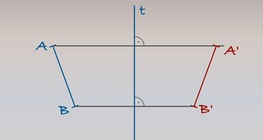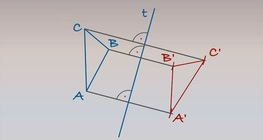Quantity: 0

Total: 0,00

# Reflecting a circle across an axis

### Reflecting a circle across an axis

Axis t and a circle with centre O and radius r are given on a particular plane. Let’s plot the mirror image of the circle.

01:38

Mathematics

Keywords

geometry, circle, reflection, editing, rotating, mathematics

Related items

### On-screen labels

Reflecting a circle across an axis

### Related items#### Reflecting a line segment across an axis

Axis t and line segment AB are given on a plane. Let’s plot the mirror image of line segment AB across t.#### Reflecting a triangle across an axis

Axis t and triangle ABC are given on a particular plane. Let’s plot the mirror image of triangle ABC.